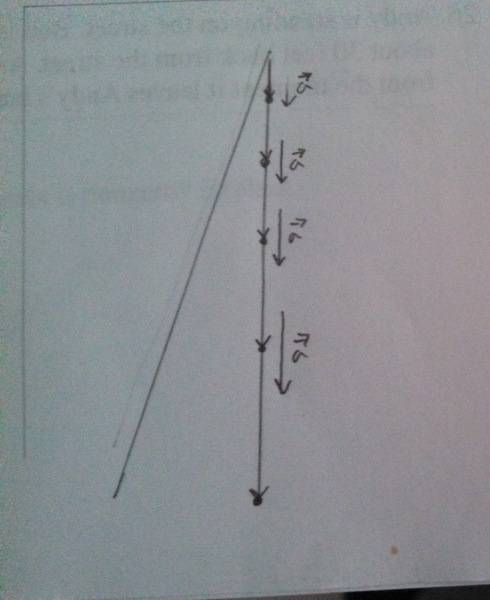# Particle Model

## Homework Statement

Draw a motion diagram for each motion described below.
-Use the particle model
-Show and label the velocity vectors

22) Galileo drops a ball from the Leaning tower of Pisa. Consider the ball's motion from the moment it leaves his hand until a microsecond before it hits the ground. Your diagram should be vertical.

## The Attempt at a SolutionThis is my stab at the problem. I believe I'm on the right track but I'm not sure how to label the velocity vectors. Do I just label them V1, V2... and so on, starting from the top?

lewando
Homework Helper
Gold Member
Your diagram shows the acceleration vector increasing. Is that what you want?

No... no it isn't. Thank you for pointing that out. Still though, what does it mean it wants me to label the velocity vectors?

lewando
Homework Helper
Gold Member
You are more likely to know what constitutes a correct motion diagram, than I (with regard to the number of points, the location of the points, labeling, whether this diagram is to show qualitative or quantitative vectors, etc.). For the points you already show, what would the velocity vectors look like?

Are you supposed to show points at equal time spacings or equal distance spacings?

We're supposed to show equal time spacing. Each dot would represent one second. The velocity vectors would increase in size as the object falls to earth do to acceleration. The arrows connecting each dot are the velocity vectors. I just don't understand what it means to label them. They're labeled in the book as V1. V2... etc. And it doesn't really go over any other sort of labeling.

lewando
Homework Helper
Gold Member
Then that sounds like all the labeling directions you need. V1 would be the velocity vector at 1 second, etc. Are you mapping this to an x-y grid? Labeling the starting height might gain you some points.